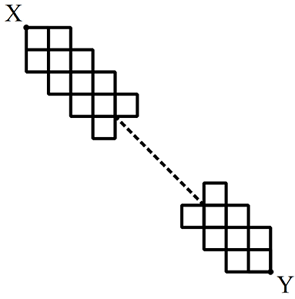# Overlapping Squares

Probability Level 4The above consists of $n$ number of $2\times2$ squares such that each adjacent $2\times2$ squares overlap each other on exactly one $1\times1$ square. And I can only move 1 unit down or 1 unit to the right at a time.

Let $M_n$ denote the total number paths for me to choose from such that I start the top left point on the top, X til I to move to the bottom right point on the bottom, Y.

Find $\displaystyle \lim_{n\to\infty} \dfrac{M_{n+1}}{M_n}$.

×